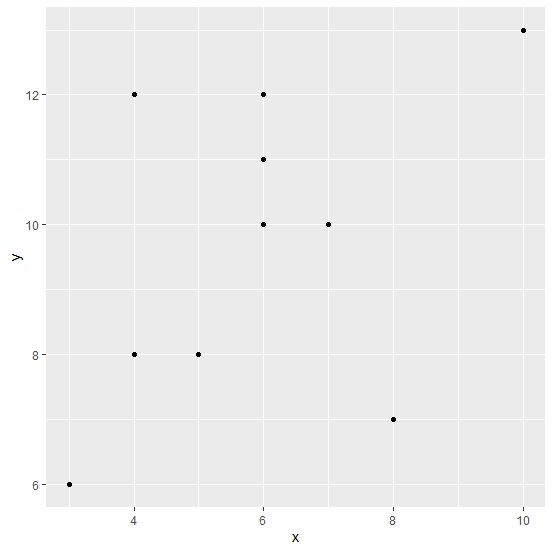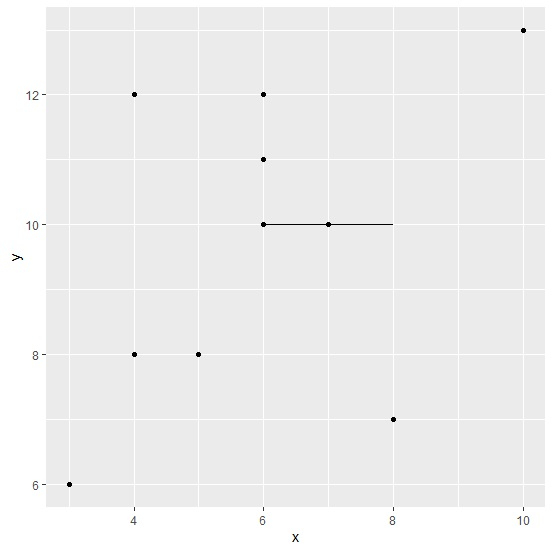# How to create horizontal line for a range of values in a plot created by using ggplot2 in R?

To display a particular part of independent variable in a plot, we might want to use a horizontal line. This will make the plot look different and get the attention of the viewer. To create a horizontal line in a plot, we can use geom_line function but we need to pass the values in a data frame format for which we want to create the horizontal line.

Consider the below data frame −

## Example

Live Demo

x<-rpois(10,6)
y<-rpois(10,8)
df<-data.frame(x,y)
df

## Output

  x y
1 6 10
2 7 17
3 5 10
4 2 10
5 6 12
6 6  9
7 4  5
8 12 5
9 5  8
10 1 8

Loading ggplot2 package and creating point chart between x and y −

## Example

library(ggplot2) ggplot(df,aes(x,y))+geom_point()

## OutputCreating the point chart between x and y by displaying a line between 6 to 8 of x when y is 10 −

## Example

ggplot(df,aes(x,y))+geom_point()+geom_line(data=data.frame(x=6:8,y=10))

## Output